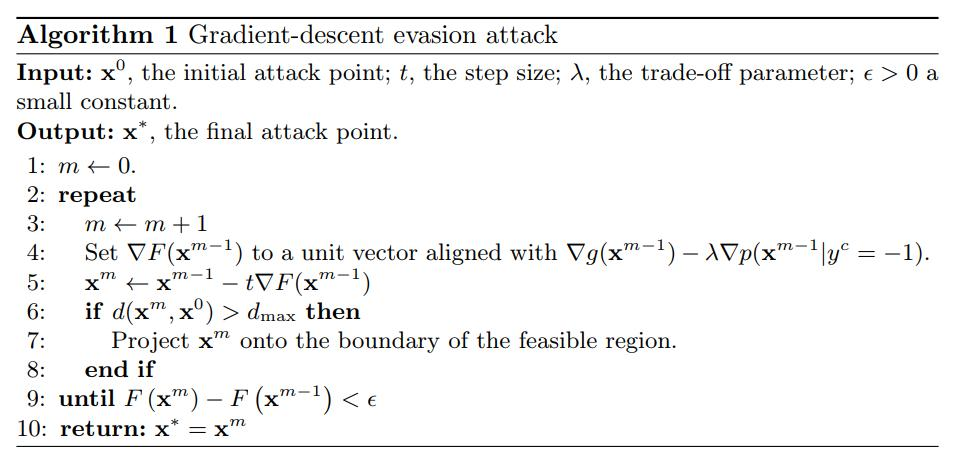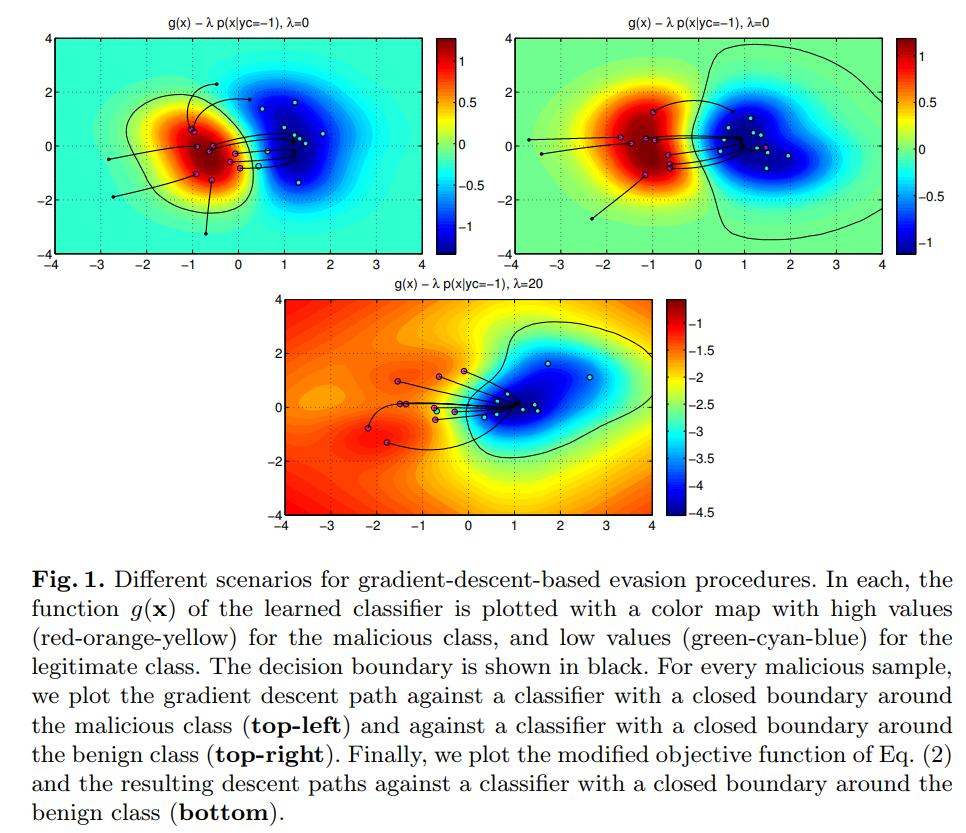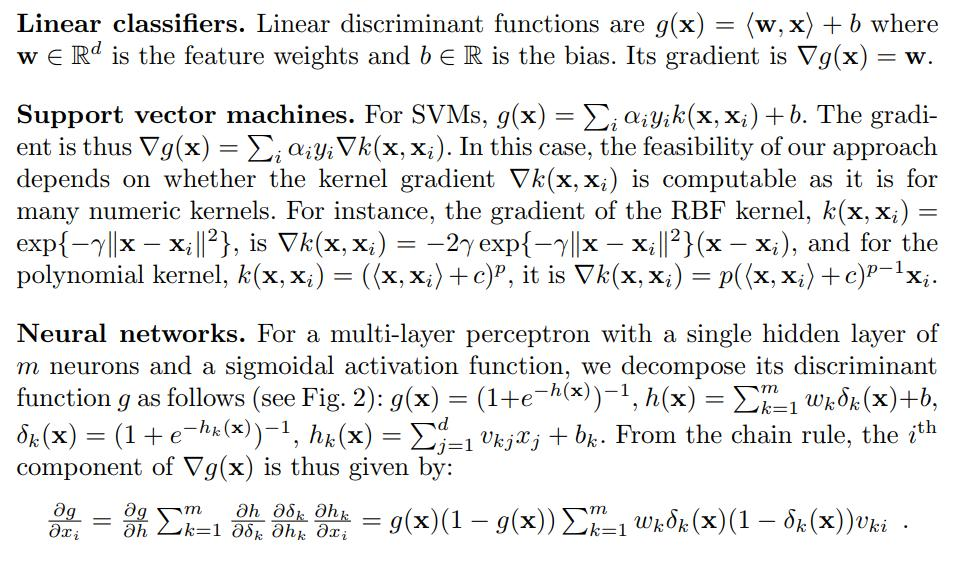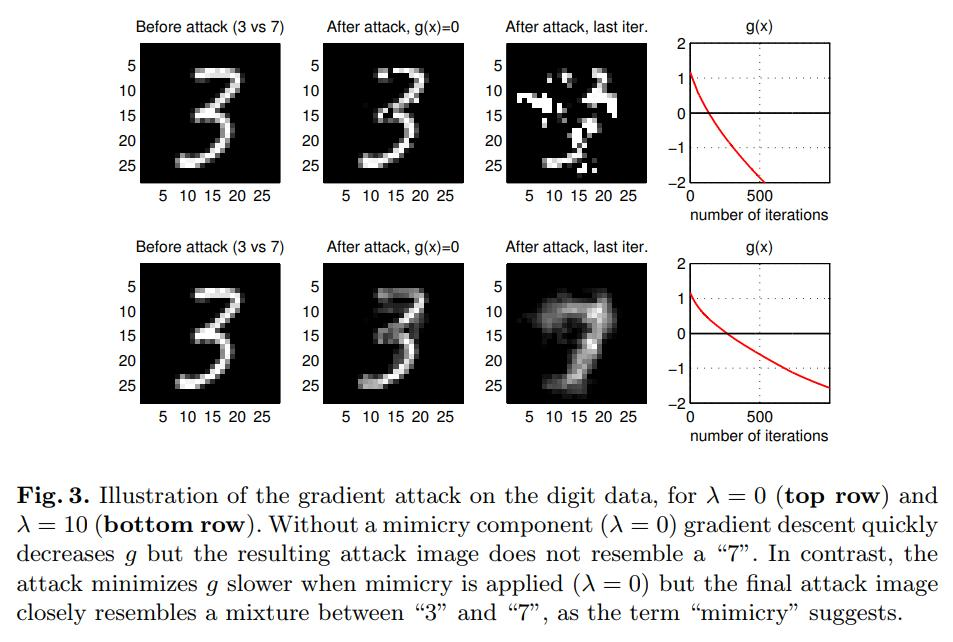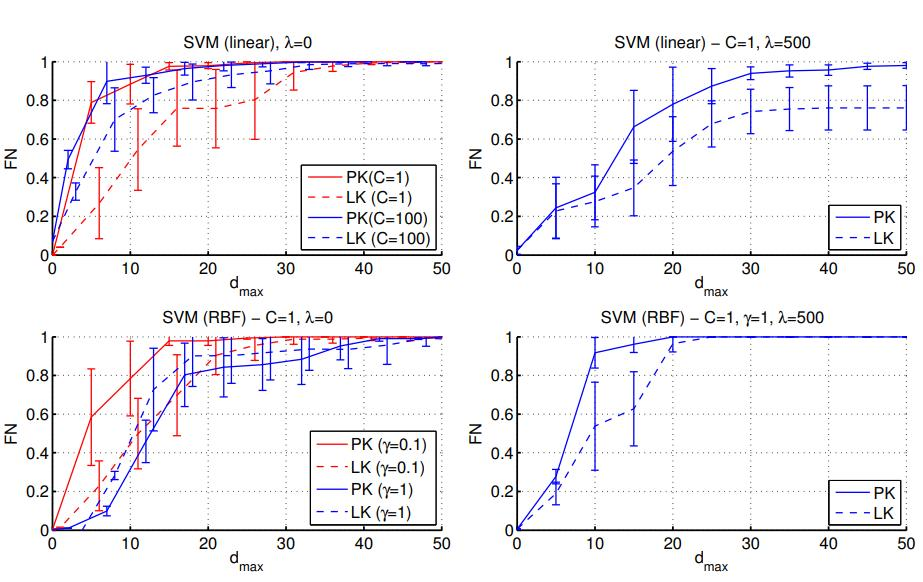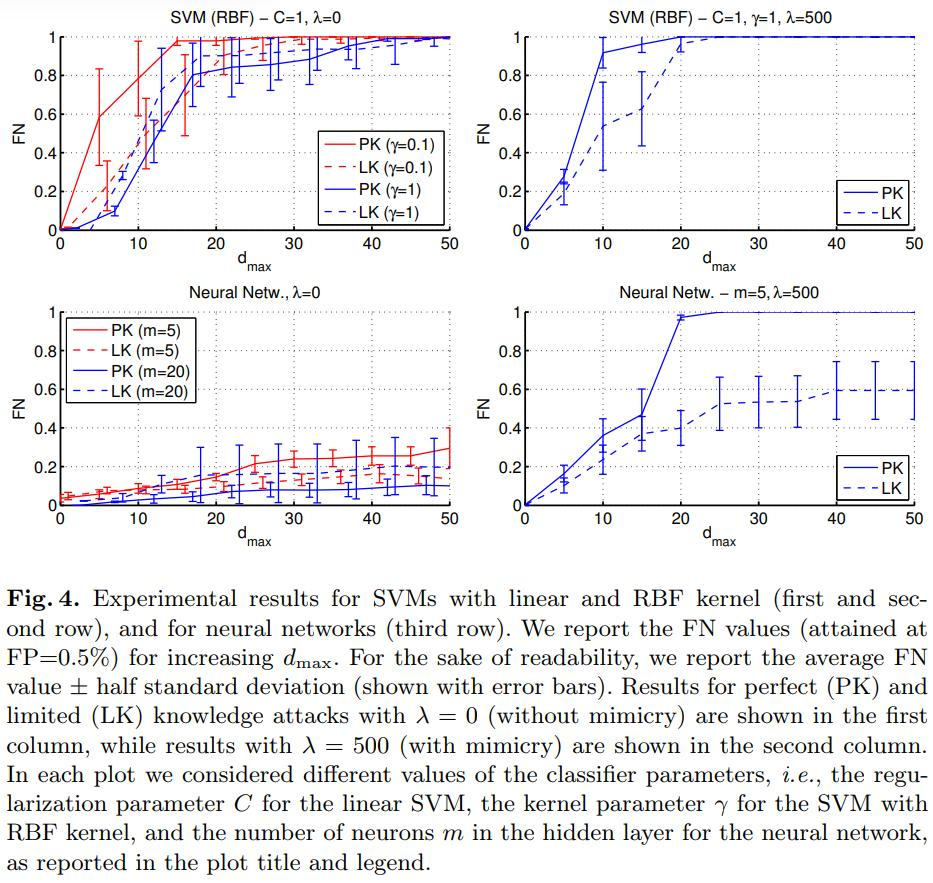# Highlights

• The authors propose an optimization method to generate adversarial examples for SVM and neural nets.
• The method has a regularization method that allows to generate adversarial examples in the black-box and white-box settings separately.
• They apply the method to malware detection in PDF files.

# Introduction

Given a classification algorithm $$f: X \mapsto Y$$ that assigns to a feature $$x \in X$$ a label $$y \in Y = \{-1,+1 \}$$, where $$-1$$ represents the legitimate class and $$-1$$ the adversarial or malicious class. The label $$y^c=f(x)$$ given by a classifier is obtained by thresholding a discriminant function $$g : X \mapsto \mathbb{R}$$, so we assume $$f(x)=-1$$ if $$g(x) < 0$$ and $$+1$$ otherwise.

• Adversary’s goal: Find a sample $$x$$ such that $$g(x)<-\epsilon$$ for every $$\epsilon>0$$. This means the sample just crosses the decision boundary. It can also be asked to find a sample where the classifier is highly confident on a wrong decision.

• Adversary’s knowledge: Depending on information about the classifier, data or training the adversary may find different strategies.

• Adversary’s capability: The authors restrict to scenarios where the adversary can only modify the test data.

## Attack scenarios

• Perfect knowledge (PK): The adversary knows everything about the classifier: architecture, data, training.

• Limited knowledge (LK): The authors assume the adversary can generate a new dataset $$D'= \{ (\hat{x}, \hat{y}) \}$$ on which to train a new classifier $$\hat{f}$$ and discriminat function $$\hat{g}$$ that approximates the original discriminant function $$g$$ of the classifier $$f$$.

## Attack strategy

For an adversarial target sample $$x^0$$, an optimal attack strategy finds sample a $$x^*$$ to minimize $$g$$ or its estimate $$\hat{g}$$, subject to a bound on its distance from $$x^0$$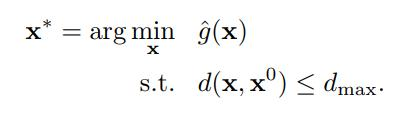But this is hard, so they regularize it as follows: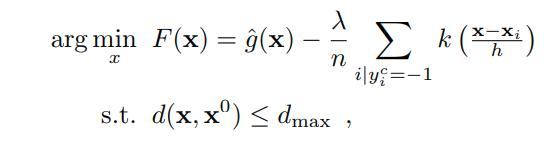They approach this via gradient descent: